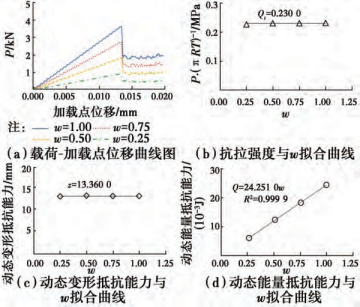News Detail

# Dynamic failure and energy dissipation of rock ring specimen based on 4D lattice spring model

1. Abstract

Rock materials are mainly brittle, with high strength, but little resistance to deformation. The introduction of pores can greatly change the properties of rock materials and improve their vibration isolation ability. However, it is difficult to analyze the large deformation of rigid rotation Angle in dynamic numerical simulation, because it needs to be approximated mathematically. The four-dimensional discrete spring element method 4DLSM solves this problem to a certain extent through the interaction of four-dimensional space. This method can deal with dynamic large deformation problems well. Firstly, the applicability of 4DLSM to a single rock ring model is verified, and the fracture morphology, dynamic deformation and energy resistance of a single rock ring model under different conditions are studied. The dynamic energy absorption characteristics and size effect of a rock ring array model with random pore structure are analyzed.

2. 4D-LSM

4D-LSM is developed on the basis of three-dimensional discrete spring element method. In DLSM, the model is composed of springs connected with discrete spherical particles, and the connecting springs between particles include normal and tangential directions. DLSM can generate different lattice structures using the same particle model, thus characterizing different macroscopic mechanical behaviors. Compared with traditional discrete methods, DLSM does not require macro parameter calibration, has half the degree of freedom of discrete element method (DEM), and is easy to parallel processing. The tangential spring in DLSM is calculated according to local strain and has rotation invariance. Based on the concept of parallel world, 4DLSM introduces the fourth dimension to construct a "four-dimensional ultra-thin film" on the basis of DLSM, and establishes the interaction relationship between the three-dimensional model and the model in the parallel world, effectively solving the Problem of Poisson's ratio limitation in the traditional LSM method. Compared with classical continuum mechanics, the discreteness of 4D-LSM makes it more suitable for simulating the failure of heterogeneous materials such as rocks or rock-like materials.

3. Verification of rock ring calculationFig.1 Rock ring models with different hole diameter ratios and failure patterns

4. Energy dissipation law of rock ringFig.2 The calculation models of different thick-diameter ratios and failure modesFig.3 Numerical simulation results of computational models with different thickness diameter ratiosFig.4 Rock ring combined computational models with different scales failure patterns.

4. Conclusion

The applicability of 4D-LSM in rock ring failure analysis is verified.

Article classification: 岩石破坏英文版
Share to：
Building 43, Beiyangyuan Campus, Tianjin University, Jinnan District, Tianjin
DiceTju@qq.com